# Deriving small unsatisfiable cores with dominators Ofer Strichman

• Slides: 27
Download presentationDeriving small unsatisfiable cores with dominators Ofer Strichman Joint work with Maya Koifman and Roman Gershman 1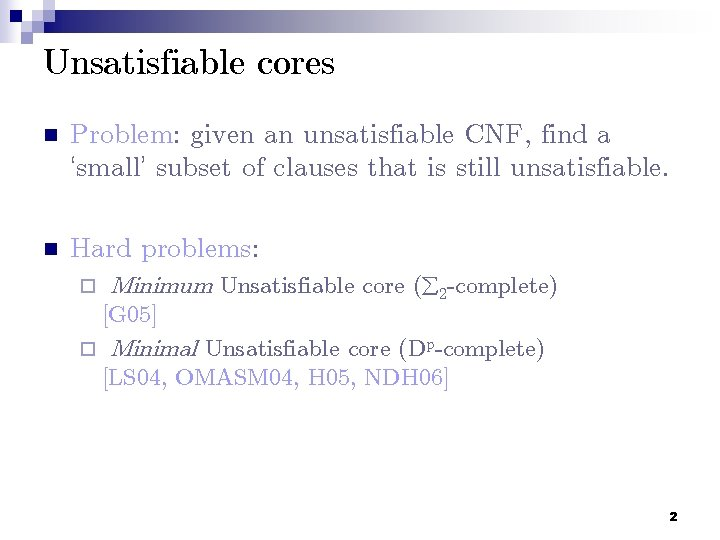Unsatisfiable cores n Problem: given an unsatisfiable CNF, find a ‘small’ subset of clauses that is still unsatisfiable. n Hard problems: ¨ Minimum Unsatisfiable core ( 2 -complete) [G 05] ¨ Minimal Unsatisfiable core (Dp-complete) [LS 04, OMASM 04, H 05, NDH 06] 2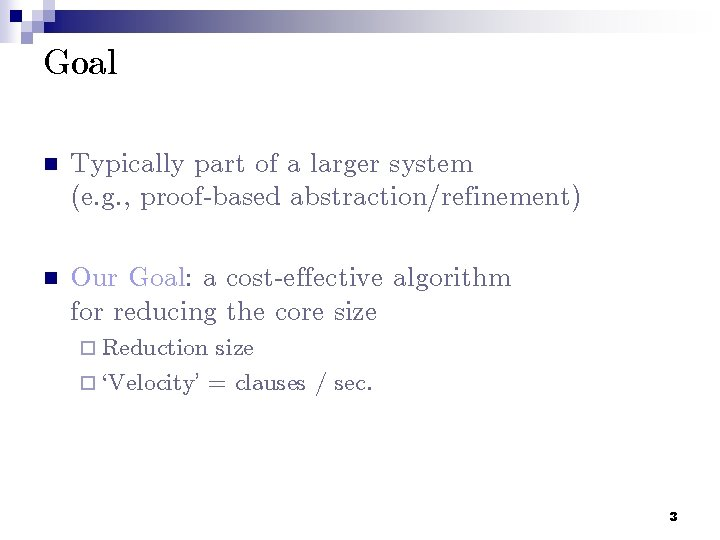Goal n Typically part of a larger system (e. g. , proof-based abstraction/refinement) n Our Goal: a cost-effective algorithm for reducing the core size ¨ Reduction size ¨ `Velocity’ = clauses / sec. 3A fixpoint approach [ZM 03] run-till-fix(φ) { Repeat forever: 1. U : = unsatcore(φ) 2. if U = φ return U; 3. φ = U } 4SAT solvers are complete resolution engines n Specifically, if the formula is unsatisfiable: ¨… they can output a resolution proof ending with the empty clause. n Our approach is based on analyzing this graph. 5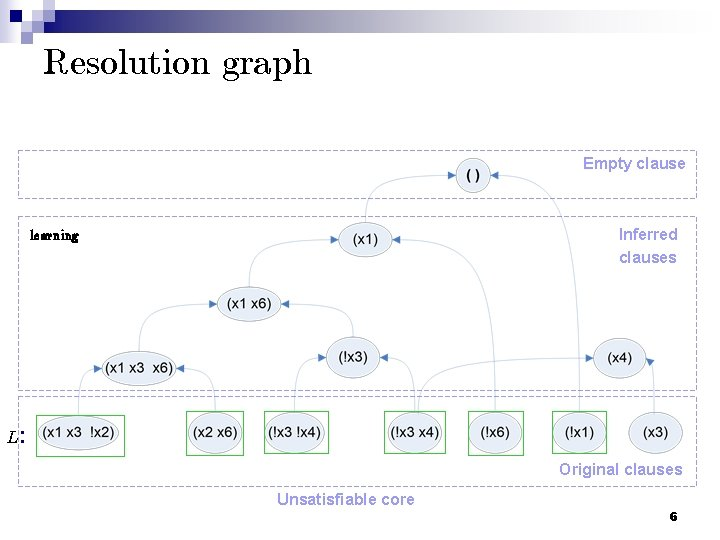Resolution graph Empty clause Inferred clauses learning L: Original clauses Unsatisfiable core 6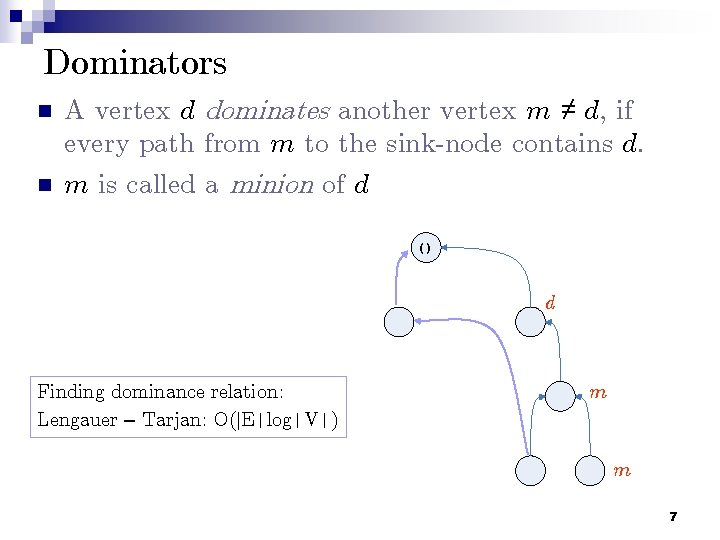Dominators n n A vertex d dominates another vertex m ≠ d, if every path from m to the sink-node contains d. m is called a minion of d () d 12 Finding dominance relation: Lengauer – Tarjan: O(|E|log|V|) m m 7Transforming the resolution graph n Observation: If d is derivable without its minions M, then M can be removed from the core. d 12 L: L’(d) 8Transforming the resolution graph n Is there a proof of node d from L’(d)? d 12 L: L’(d) 9An alternative proof. n Suppose we have an alternative proof of d from L’(d): d 12 L: L’(d) 10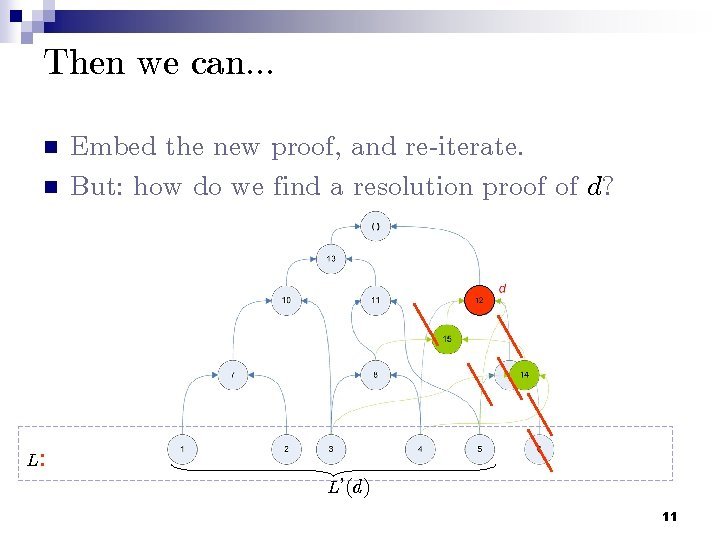Then we can. . . n n Embed the new proof, and re-iterate. But: how do we find a resolution proof of d? 12 L: L’(d) 11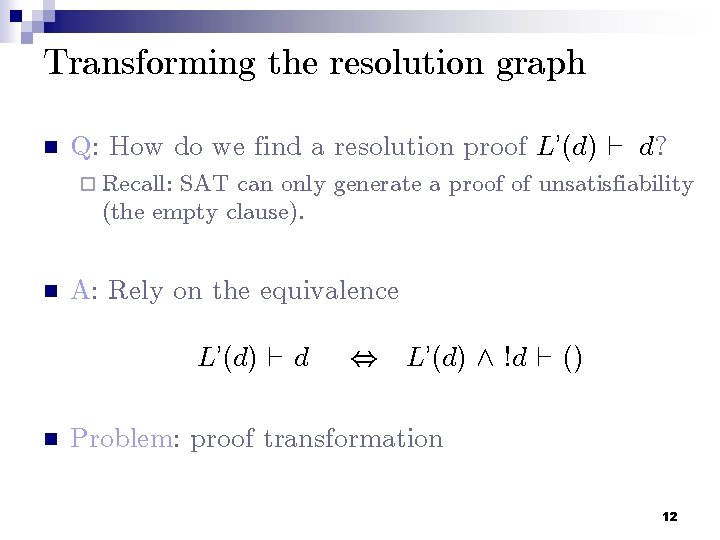Transforming the resolution graph n Q: How do we find a resolution proof L’(d) ` d? ¨ Recall: SAT can only generate a proof of unsatisfiability (the empty clause). n A: Rely on the equivalence L’(d) ` d n , L’(d) ^ !d ` () Problem: proof transformation 12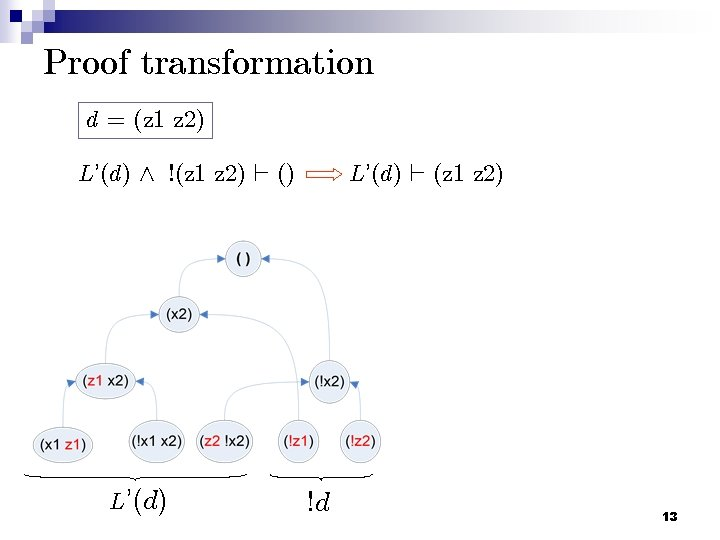Proof transformation d = (z 1 z 2) L’(d) ^ !(z 1 z 2) ` () L’(d) ` (z 1 z 2) !d 13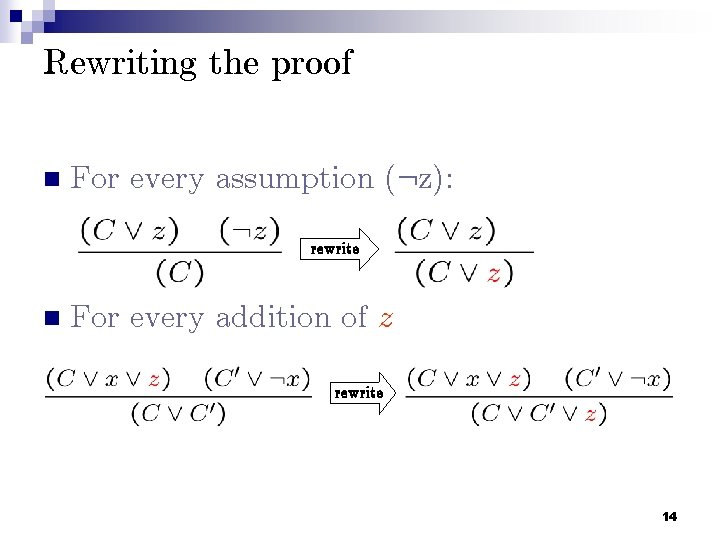Rewriting the proof n For every assumption (: z): rewrite n For every addition of z rewrite 14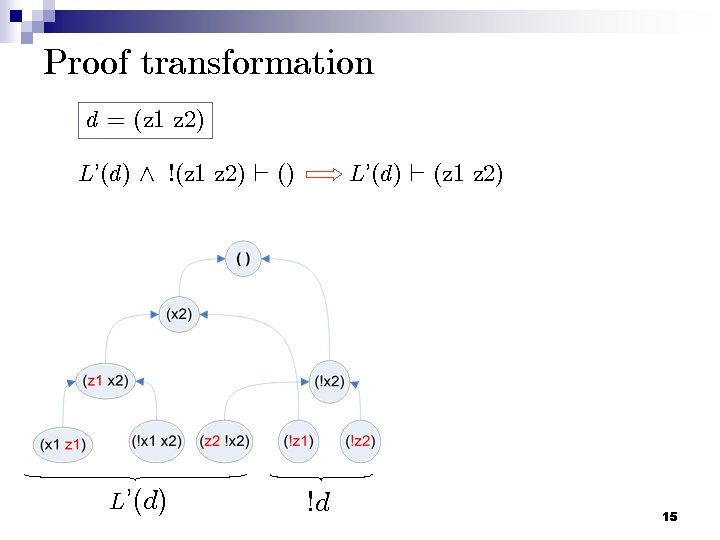Proof transformation d = (z 1 z 2) L’(d) ^ !(z 1 z 2) ` () L’(d) ` (z 1 z 2) !d 15Proof transformation L’(d) ^ !(z 1 z 2) ` () L’(d) ` (z 1 z 2) !d 16Proof transformation L’(d) ^ !(z 1 z 2) ` () L’(d) ` (z 1 z 2) !d 17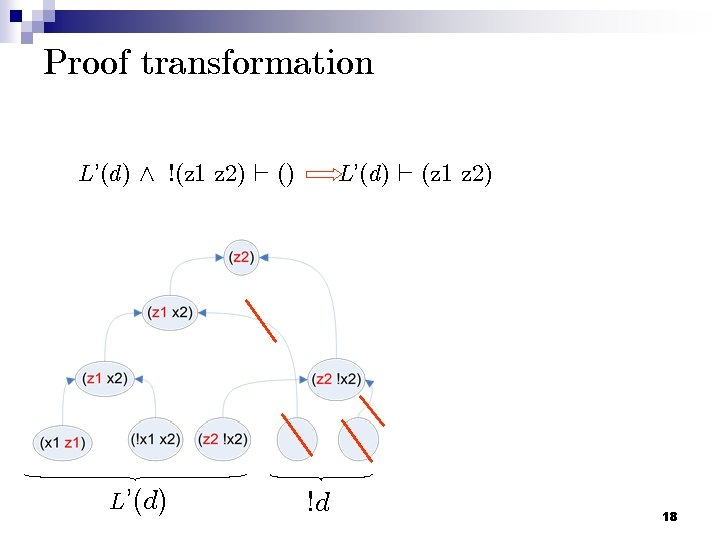Proof transformation L’(d) ^ !(z 1 z 2) ` () L’(d) ` (z 1 z 2) !d 18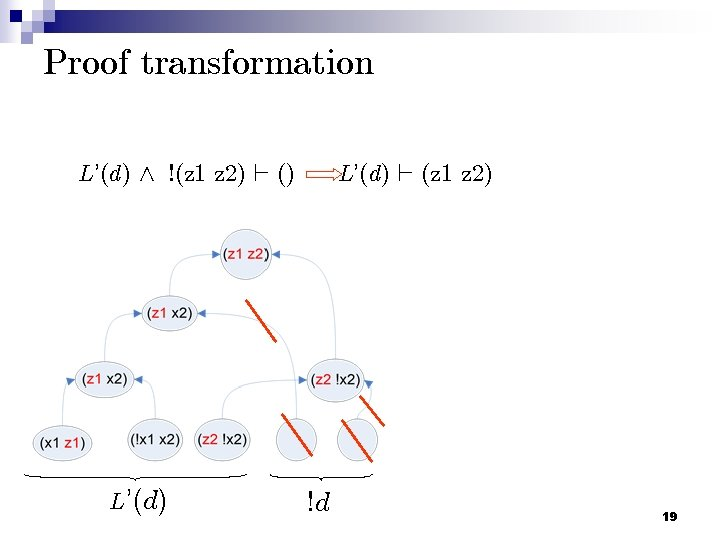Proof transformation L’(d) ^ !(z 1 z 2) ` () L’(d) ` (z 1 z 2) !d 19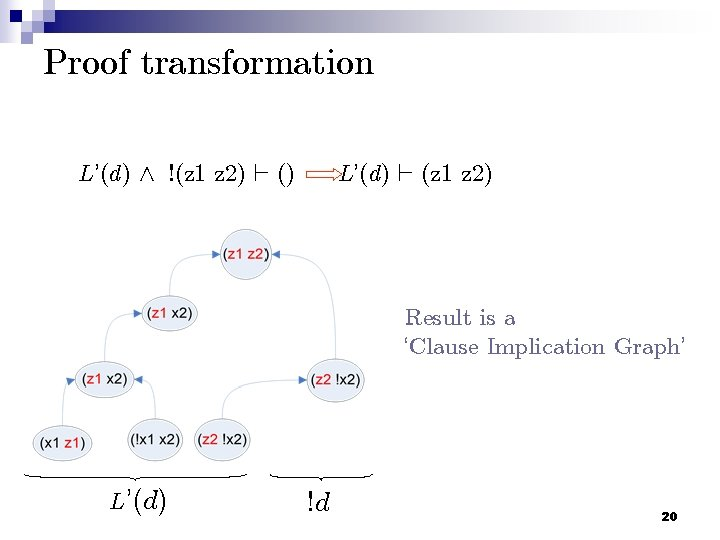Proof transformation L’(d) ^ !(z 1 z 2) ` () L’(d) ` (z 1 z 2) Result is a ‘Clause Implication Graph’ L’(d) !d 20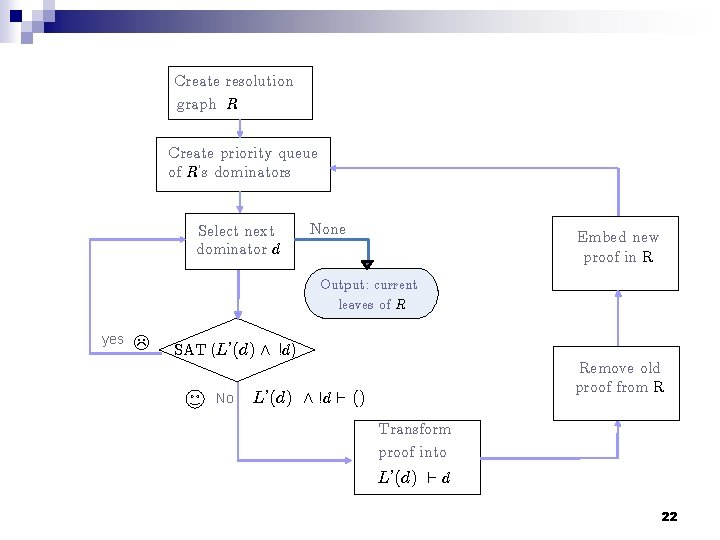Create resolution graph R Create priority queue of R’s dominators Select next dominator d None Embed new proof in R Output: current leaves of R yes SAT (L’(d) ^ !d) No Remove old proof from R L’(d) ^ !d ` () Transform proof into L’(d) ` d 22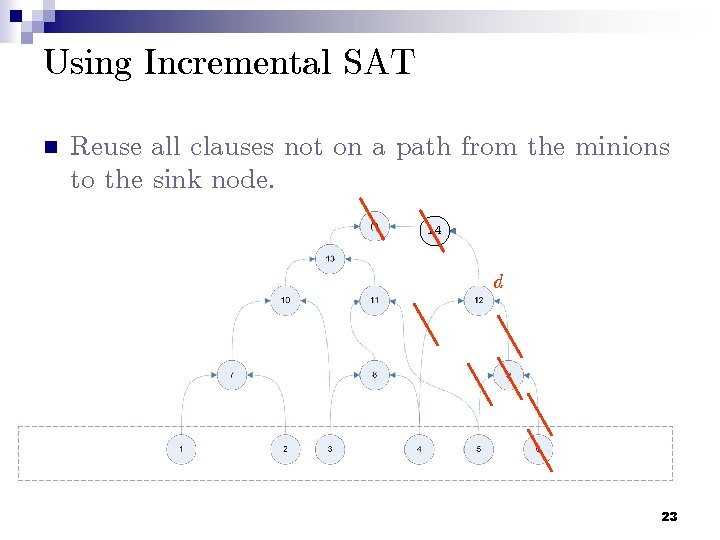Using Incremental SAT n Reuse all clauses not on a path from the minions to the sink node. 14 d 23Results – Accumulated # removed clauses 71 industrial benchmarks (timeout – 1 hour) 24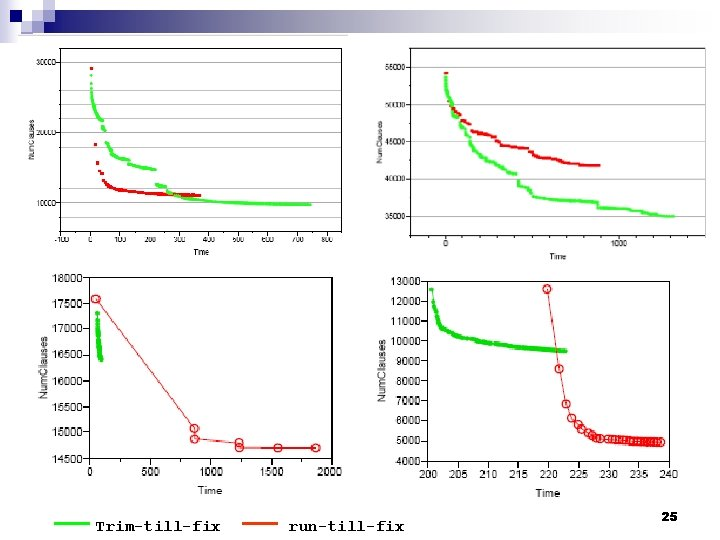Trim-till-fix run-till-fix 2571 industrial benchmarks (timeout – 1 hour) Trim-till-fix Core reduction: Run-till-fix 26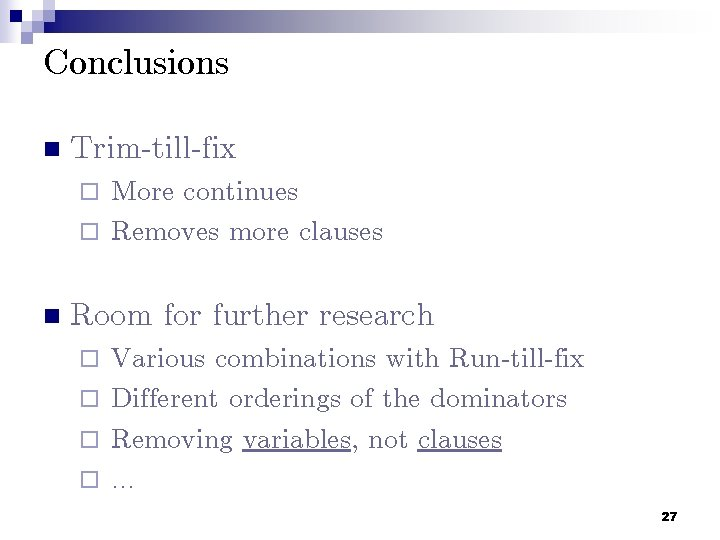Conclusions n Trim-till-fix ¨ More continues ¨ Removes more clauses n Room for further research ¨ Various combinations with Run-till-fix ¨ Different orderings of the dominators ¨ Removing variables, not clauses ¨ … 27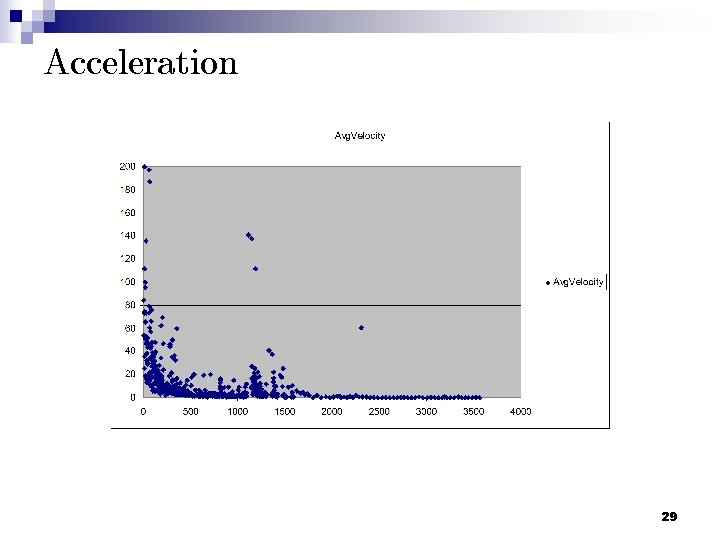Acceleration 29